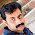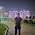## Electronic Devices Objective Questions:

 The action of JFET in its equivalent circuit can be represented as a
A. Current controlled Current source
B. Current controlled Voltage source
C. Voltage controlled Voltage source
D. Voltage controlled Current source

 A change in the value of the emitter resistance Re in a differential amplifier
A. Affects the difference mode gain Ad
B. Affects the common mode gain Ac
C. Affects both Ad and Ac
D. Does not effect either Ad and Ac

 Generally, the gain of a transistor amplifier falls at high frequencies due to the
A. Internal Capacitance of the device
B. Coupling capacitor at the input
C. Skin effect
D. Coupling capacitor at the output

 In a common emitter, unbypassed resistor provides
A. Voltage shut feedback
B. Current series feedback
C. Negative voltage feedback
D. Positive current feedback

 A constant current signal across a parallel RLC circuits gives an o/p of 1.4V at the signal frequency of 3.89kHz. At the frequency of 4 kHz, the o/p voltage will be
A. 1 V
B. 2 V
C. 1.4 V
D. 2.8 V

 Class AB operation is often used in power (large signal) amplifiers in order to
A. Get maximum effeciency
B. Remove even harmonics
C. Overcome a crossover distortion
D. Reducing collector dissipation

 The bandwidth of an RF tuned amplifier is dependent on
A. Q-factor of the tuned O/P circuit
B. Q-factor of the tuned I/P circuit
C. Quiescent operating point
D. Q-factor of the O/P and I/P circuits as well as quiescent operating point

Most of the linear ICs are based on the two-transistor differential amplifier because of its
A. Input voltage dependent linear transfer characteristics
B. High voltage gain
C. High input resistance
D. High CMMR

 Negative feedback in an amplifier
A. Reduces gain
B. Increase frequency and phase distortion
C. Reduces bandwidth
D. Increase Noise

10] A dc power supply has no-load voltage of 30V and a full-load voltage of 25V at full-load current of 1A. Its output resistance and load regulation respectively are
A. 5Ω & 20%
B. 25Ω & 20%
C. 5Ω &16.7%
D. 25Ω & 16.7%
6:39 PM

1.1.